Win up to 100% scholarship on Aakash BYJU'S JEE/NEET courses with ABNAT Win up to 100% scholarship on Aakash BYJU'S JEE/NEET courses with ABNAT

# Electrostatics

## What Is Electrostatics?

The study of stationary electric charges at rest is known as electrostatics. An electroscope is used to detect the charge on a body. A pith ball electroscope is used to detect a charge and to know the nature of the charge. Gold leaf electroscope, which was invented by Abraham Bennet, detects a charge, the nature of the charge and determines the quantity of the charge.

### Conductors, Insulators, and Semiconductors

• A body in which electric charge can easily flow through is called a conductor (For example, metals).
• A body in which electric charge cannot flow is called an insulator or dielectric. (For example, glass, wool, rubber, plastic, etc.)
• Substances which are intermediate between conductors and insulators are called semiconductors. (For example, silicon, germanium, etc.)

Dielectric Strength: It is the minimum field intensity that should be applied to break down the insulating property of the insulator.

• Dielectric strength of air = 3 x 106 V/m
• Dielectric strength of Teflon = 60 × 106 Vm–1

The maximum charge a sphere can hold depends on the size and dielectric strength of the medium in which the sphere is placed.

1. The maximum charge density of a sphere of radius ‘R’ in terms of electric intensity E at a distance in free space is Eε0(R/r)2.
2. When the electric field in the air exceeds its dielectric strength, air molecules become ionised and are accelerated by fields and the air becomes conducting.

Download Free PDFs of Daily Practice Problems and Worksheets for Electrostatics Concept

### Surface Charge Density σ

The charge per unit area of a conductor is defined as surface charge density.

$$\begin{array}{l}\sigma = \frac{q}{A}=\frac{total\,\,charge}{area}\end{array}$$
. When A = 1 m2, then σ = q.

Its unit is coulomb/metre, and its dimensions are ATL–2. It is used in the formula for the charged disc, charged conductor, an infinite sheet of charge etc.  The surface charge density depends on the shape of the conductor and the presence of other conductors and insulators in the vicinity of the conductor.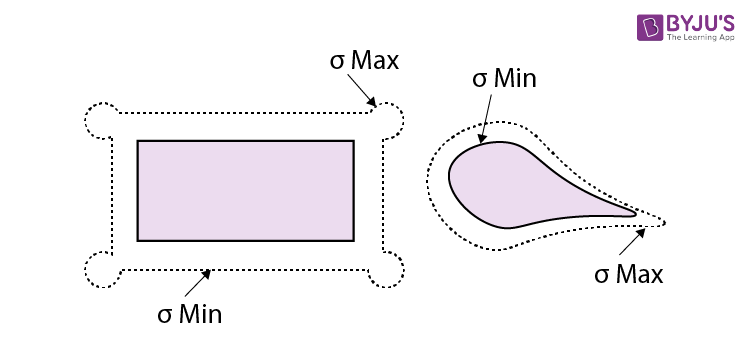1. $$\begin{array}{l}\sigma\alpha \frac{1}{r^{2}}\;i.e.\frac{\sigma_1}{\sigma_2} = \frac{r_{2}^{2}}{r_{1}^{2}}\end{array}$$
2. σ is maximum at pointed surfaces, and for plane surfaces, it is minimum.
3. Surface charge density is maximum at the corners of rectangular laminas and at the vertex of the conical conductor.

## Electric Flux

The number of electric lines of force crossing a surface normal to the area gives electric flux ΦE.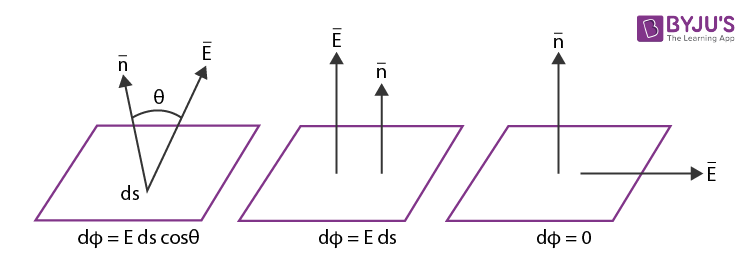The electric flux through an elementary area ds is defined as the scalar product of area and field.

dΦE = Eds cos θ

Or,

$$\begin{array}{l}\phi _E = \int \vec{E}.\vec{ds}\end{array}$$
• Electric Flux will be maximum when the electric field is normal to the area (dΦ = Eds)
• Electric Flux will be minimum when the field is parallel to the area (dΦ = 0)
• For a closed surface, outward flux is positive and inward flux is negative

## Electric Potential (V)

The electric potential at a point in a field is the amount of work done in bringing a unit +ve charge from infinity to the point. It is equal to the electric potential energy of unit + ve charge at that point.

• It is a scalar quantity.
• The SI unit is volts.

The electric potential at a distance ‘d’ due to a point charge q in air or vacuum is

$$\begin{array}{l}V =\frac{1}{4\pi {{\varepsilon }_{0}}}.\frac{q}{d}\end{array}$$

Electric potential (V)

$$\begin{array}{l}= -\int{\vec{E}.\vec{d}x}\end{array}$$

$$\begin{array}{l}\vec{E}=-\frac{dv}{dx}\end{array}$$
(or) V = Ed

A positive charge in a field moves from high potential to low potential, whereas an electron moves from low potential to high potential when left free. Work done in moving a charge q through a potential difference V is W = q V joule

• Gain in the kinetic energy:
$$\begin{array}{l}\frac{1}{2}m{{v}^{2}}=qV\end{array}$$
• Gain in the velocity:
$$\begin{array}{l}v\,\,=\sqrt{\frac{2qV}{m}}\end{array}$$

## Equipotential Surface

A surface on which all points are at the same potential is called an equipotential surface.

1. The electric field is perpendicular to the equipotential surface.
2. Work done in moving a charge on the equipotential surface is zero.

### In the Case of a Hollow Charged Sphere

1. Intensity at any point inside the sphere is zero.
2. Intensity at any point on the surface is the same, and it is maximum
$$\begin{array}{l}\frac{1}{4\pi {{\varepsilon }_{0}}}.\frac{q}{{{r}^{2}}}\end{array}$$
3. Outside the sphere
$$\begin{array}{l}\frac{1}{4\pi {{\varepsilon }_{0}}}.\frac{q}{{{d}^{2}}}\end{array}$$
i.e.d = distance from the centre. It behaves as if the whole charge is at its centre.

Electric field intensity in vector form

$$\begin{array}{l}\vec{E}=\,\,\;\frac{1}{4\pi {{\varepsilon }_{0}}}.\frac{q}{{{d}^{3}}}\vec{d} \;or\; \vec{E}\,\,=\,\,\;\;\frac{1}{4\pi {{\varepsilon }_{0}}}.\frac{q}{{{d}^{3}}}\hat{d}\end{array}$$

The resultant electric field intensity obeys the principle of superposition.

$$\begin{array}{l}\vec{E}\,\,=\,\,{{\vec{E}}_{1\,\,}}+{{\vec{E}}_{2}}+{{\vec{E}}_{3}}+……………\end{array}$$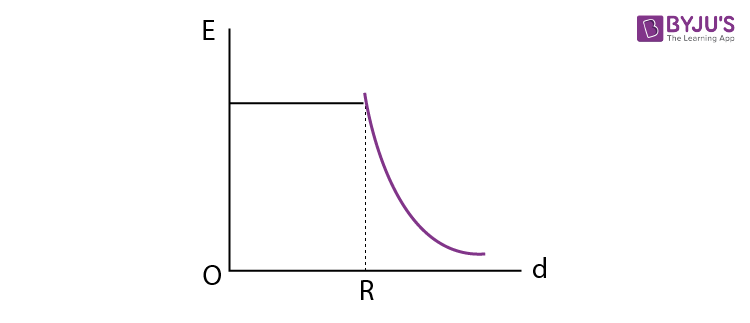### In the Case of Solid Charged Sphere

The potential at any point inside the sphere is the same as that at any point on its surface.

$$\begin{array}{l}V = \frac{1}{4\pi {{\varepsilon }_{0}}}.\frac{q}{r}\end{array}$$

It is an equipotential surface. Outside the sphere, the potential varies inversely as the distance of the point from the centre.

$$\begin{array}{l}V = \frac{1}{4\pi {{\varepsilon }_{0}}}.\frac{q}{d}\end{array}$$

Note: Inside a non-conducting charged sphere electric field is present.

Electric intensity inside the sphere

$$\begin{array}{l}E = \frac{1}{4\pi {{\varepsilon }_{0}}}.\frac{Q}{{{R}^{3}}}d\end{array}$$

Here, d is the distance from the centre of the sphere and E d is inside the sphere but falls off like 1/doutside the sphere.

## Electron Volt

This is the unit of energy in particle physics and is represented as eV.

• 1 eV = 1.602 x 10‑19 J.

### Charged Particles in Electric Field

When a positive test charge is fired in the direction of an electric field,

• It accelerates
• Its kinetic energy increases
• Its potential energy decreases

A charged particle of mass m carrying a charge q and falling through a potential V acquires a speed of

$$\begin{array}{l}\sqrt{2Vq/m}\end{array}$$
.

## Electric Dipole

Two equal and opposite charges separated by a constant distance is called an electric dipole.

$$\begin{array}{l}\vec{P}=q.2\bar{l}\end{array}$$

### Dipole Moment

It is the product of one of the charges and the distance between the charges. It is a vector directed from the negative charge towards the positive charge along the line joining the two charges.

The torque acting on an electric dipole placed in a uniform electric field is given by the relation,

$$\begin{array}{l}\vec{\tau }=\vec{P}\text{ }x\text{ }\vec{E}\ \text{ }i.e.,\ \tau =PE\sin \theta,\end{array}$$
$$\begin{array}{l}\text{where}\ \theta\ \text{is the angle between}\ \vec{P}\ \text{and}\ \vec{E}.\end{array}$$

⇒ The electric intensity (E) on the axial line at a distance’d’ from the center of an electric dipole is

$$\begin{array}{l}E=\frac{1}{4\pi {{\varepsilon }_{0}}}\cdot \frac{2Pd}{{{({{d}^{2}}-{{l}^{2}})}^{2}}}\end{array}$$
and on equatorial line, the electric intensity (E)
$$\begin{array}{l}=\frac{1}{4\pi {{\varepsilon }_{0}}}\cdot \frac{P}{{{({{d}^{2}}+{{l}^{2}})}^{3/2}}}\end{array}$$
.

⇒ For a short dipole, i.e., if l2 << d2, then the electric intensity on the equatorial line is given by

$$\begin{array}{l}E =\frac{1}{4\pi {{\varepsilon }_{0}}}\cdot \frac{P}{{{d}^{3}}}\end{array}$$
.

⇒ The potential due to an electric dipole on the axial line is

$$\begin{array}{l}V =\frac{1}{4\pi {{\varepsilon }_{0}}}\cdot \frac{P}{({{d}^{2}}-{{l}^{2}})}\end{array}$$
, and at any point on the equatorial line, it is zero.

When two unlike equal charges, +Q and –Q, are separated by a distance

• The net electric potential is zero on the perpendicular bisector of the line joining the charges.
• The bisector is an equipotential and zero potential line.
• Work done in moving a charge on this line is zero.
• Electric intensity at any point on the bisector is perpendicular to the bisector.
• Electric intensity at any point on the bisector parallel to the bisector is zero.

### Combined Field Due to Two Point Charges

#### Due to two similar charges

If charges q1 and q2 are separated by a distance ‘r’, a null point (where the resulting field intensity is zero) is formed on the line joining these two charges.

1. The null point is formed within the charges.
2. The null point is located nearer to the weak charge.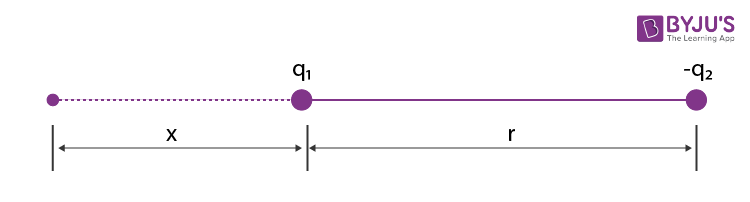If x is the distance of a null point from q1,

(weak charge) then

$$\begin{array}{l}\frac{{{\text{q}}_{\text{1}}}}{{{\text{x}}^{\text{2}}}}\,\,=\,\,\frac{{{q}_{2}}}{{{(r-x)}^{2}}}\end{array}$$

$$\begin{array}{l}\Rightarrow \,\,x\,\,=\,\,\frac{r}{\sqrt{{{q}_{2}}/{{q}_{1}}\,}\,\,+1}\end{array}$$
. Here, q1 and q2 are like charges.

#### Due to two dissimilar charges

1. If q1 and q2 are unlike charges, then a null point is formed on the line joining two charges.
2. The null point is formed outside the charges.
3. The null point is from a nearer weak charge.
4. x is the distance of null point from q1(weak charge) then
$$\begin{array}{l}\frac{{{\text{q}}_{\text{1}}}}{{{\text{x}}^{\text{2}}}}\,\,=\,\,\frac{{{q}_{2}}}{{{(r+x)}^{2}}}\end{array}$$
$$\begin{array}{l}\Rightarrow \,\,x\,\,=\,\,\frac{r}{\sqrt{{{q}_{2}}/{{q}_{1}}\,}\,\,-1}\end{array}$$
$$\begin{array}{l}\text{In the above formulae }\ {{q}_{2}}/{{q}_{1}}\ \text{is numerical ratio of charges.}\end{array}$$

#### Zero potential points due to two charges

• If two unlike charges, q1 and q2, are separated by a distance ‘r’, the net potential is zero at two points on the line joining them.
• One in between them and the other outside the charges.
• Both points are nearer to the weak charge (q1).
$$\begin{array}{l}\frac{{{\text{q}}_{\text{1}}}}{{{\text{x}}^{{}}}}\,\,=\,\,\frac{{{q}_{2}}}{(r-x)}\end{array}$$
(for point 1, with in the charges)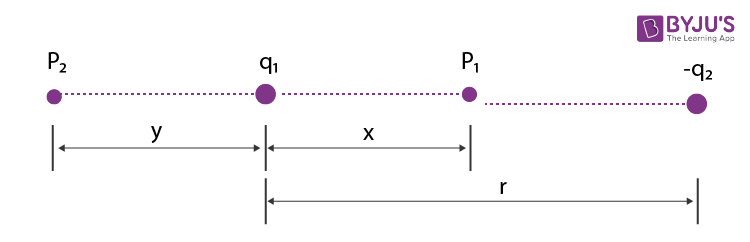$$\begin{array}{l}\frac{{{\text{q}}_{\text{1}}}}{{{\text{y}}^{{}}}}\,\,=\,\,\frac{{{q}_{2}}}{(r+y)}\end{array}$$
(for point 2, outside the charges). Here, q2 is the numerical value of a strong charge.

$$\begin{array}{l}\Rightarrow \,\,x\,\,=\,\,\frac{r}{\frac{{{q}_{2}}}{{{q}_{1}}}+1}\;\; ; y\,\,=\,\,\frac{r}{\frac{{{q}_{2}}}{{{q}_{1}}}-1}\end{array}$$

Due to two similar charges zero potential point is not formed.

## Electric Lines of Force

The line of force is the path along which a unit +ve charge accelerates in the electric field. The tangent at any point to the line of force gives the direction of the field at that point.

### Properties of Electric Lines of Force

• Two lines of force never intersect.
• The number of lines of force passing normally through a unit area around a point is numerically equal to E, the strength of the field at the point.
• Lines of force always leave or end normally on a charged conductor.
• Electric lines of force can never be closed loops.
• Lines of force have a tendency to contract longitudinally and exert a force of repulsion on one another laterally.
• If in a region of space, there is no electric field, there will be no lines of force. Inside a conductor, there cannot be any line of force.

The number of lines of force passing normally through a unit area around a point is numerically equal to E.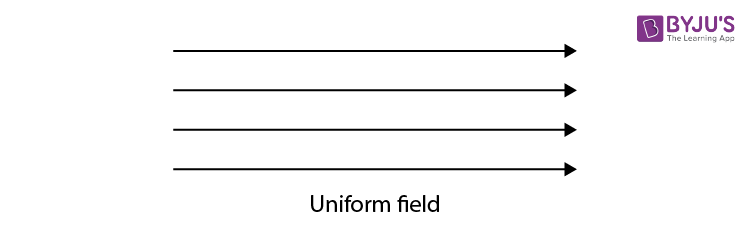In a uniform field, lines of force are parallel to one another.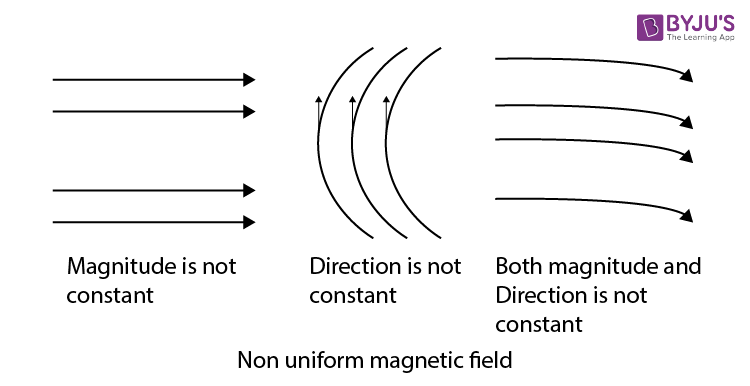Difference between electric lines of force and magnetic lines of force

• Electric lines of force never form closed loops, while magnetic lines are always closed loops.
• Electric lines of force do not exist inside a conductor, but magnetic lines of force may exist inside a magnetic material.

## Recommended Video Lessons

### Electrostatics – Electric Field Lines and Electric Flux### Electrostatics – Electric Field and Electric Dipole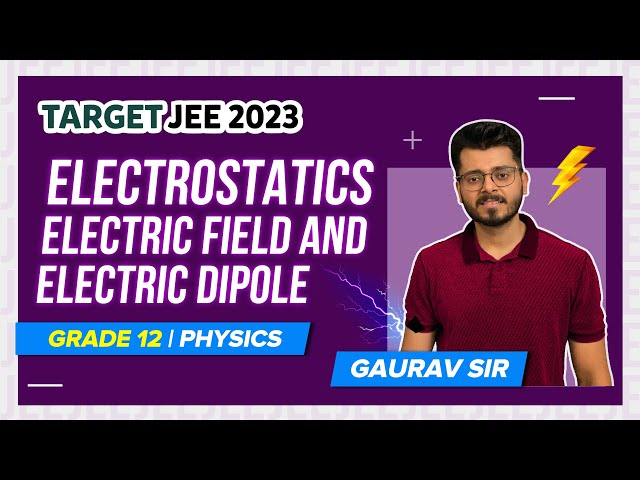### Electrostatics – Coulomb’s Law and Superposition Principle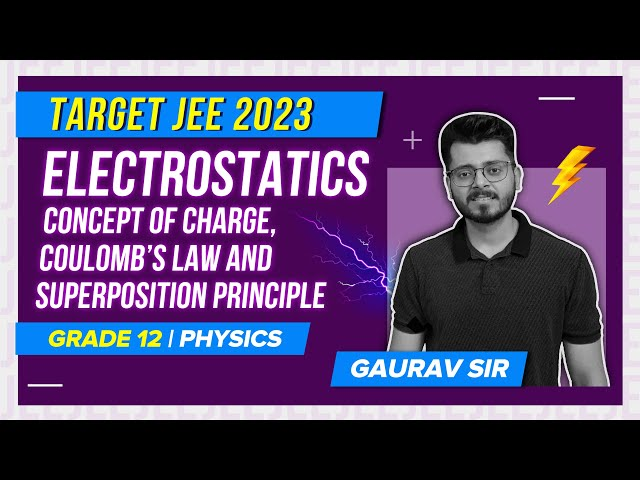### 30 Must-Do Questions of Electrostatics for JEE Main### Electrostatics and Capacitor – Important Topics### Electrostatics and Capacitor – Important Questions## Electrostatics Problems and Solutions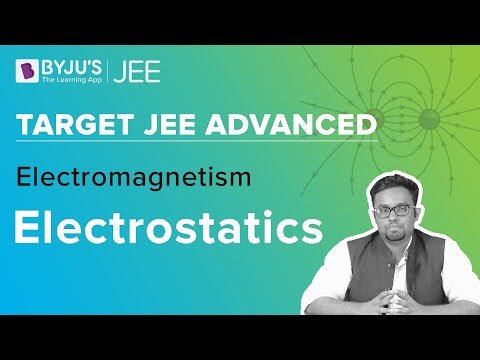## Electrostatics JEE Main Important Questions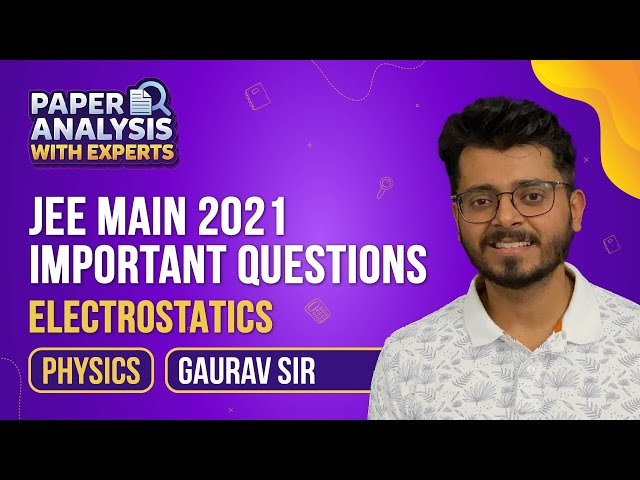## Electrostatics – JEE Advanced Concepts & Questions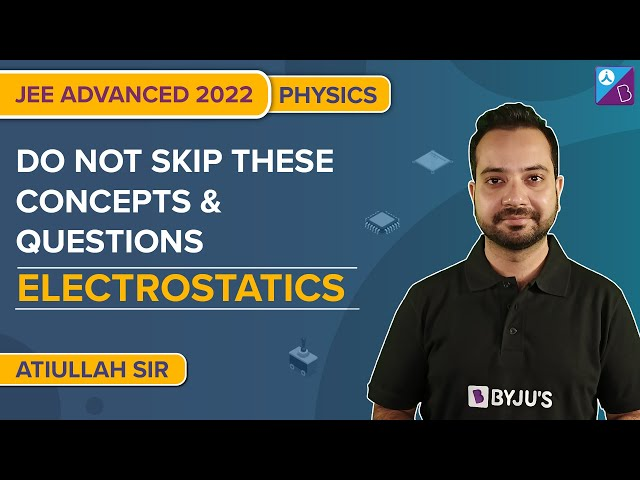## Frequently Asked Questions on Electrostatics

Q1

### Is the electrostatic force between the two-point charges a central force?

Yes, the electrostatic force between two point charges always acts along the line joining the two charges. Hence, it is a central charge.

Q2

### When is the electrostatic force between two given charges maximum for a given separation between them?

The electrostatic force between two given charges separated by a certain distance is maximum when the charges are in air or vacuum.

Q3

### Does electrostatic force obey Newton’s third law?

Yes. If charge q1 exerts a force on q2, then q2 exerts an equal and opposite force on q1.

Q4

### What is charging by conduction?

Charging by conduction is a method by which a conductor is charged by bringing a charged body in contact with the conductor.